## AND Function

The AND function is a premade function in Google Sheets, which returns TRUE or FALSE based on two or more conditions.

It is typed `=AND` and takes two or more conditions.

=AND([logical_expression1], [logical_expression2, ...])

The conditions are referred to as `[logical_expression1]`, `[logical_expression2]`, and so on.

The conditions can check things like:

• If a number is greater than another number `>`
• If a number is smaller than another number `<`
• If a number or text is equal to something `=`

Note: The AND function is often used together with the IF function.

## Example AND Function

Check if the Pokemon type is fire and has speed greater than 70:

The function returns "TRUE" or "FALSE".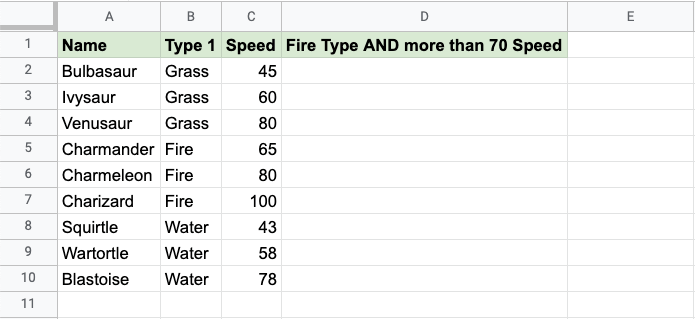Copy Values

Example AND function, step by step:

1. Select the cell `D2`
2. Type `=AND`
3. Click the AND command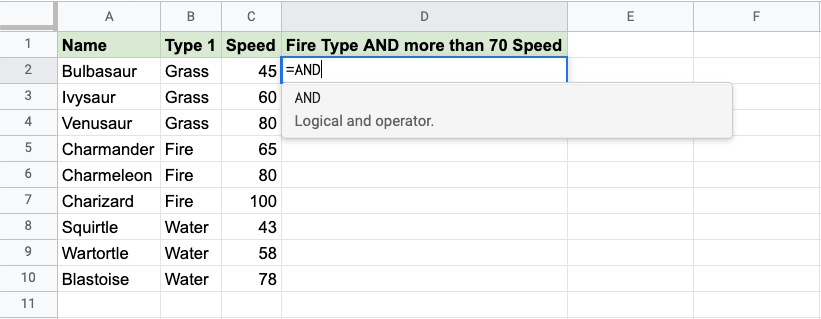1. Specify the first condition `B2="Fire"`
2. Type `,`
3. Specify the second condition `C2>70`
4. Hit enter

Note: You can add more conditions by repeating steps 5-6 before hitting enter.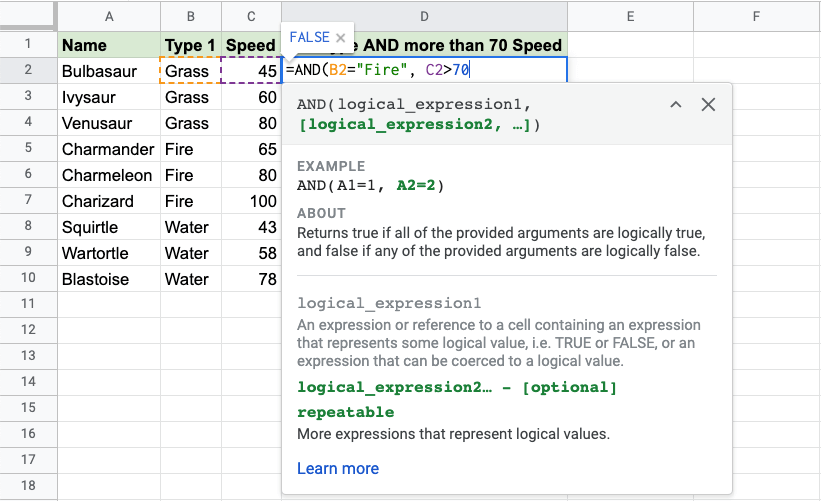Since the value in cell `B2` is not "Fire" the first condition is FALSE.

Since the value in cell `C2` is less than 70 the second condition is also FALSE.

All conditions need to be TRUE for the AND function to return TRUE.

Note: Text values needs to be in quotes: " "

The function can be repeated with the filling function for each row to perform the same check for each Pokemon: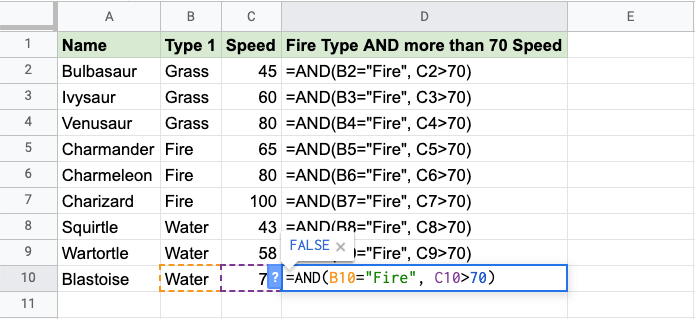Now, each row has a check for Fire Type and Speed greater than 70: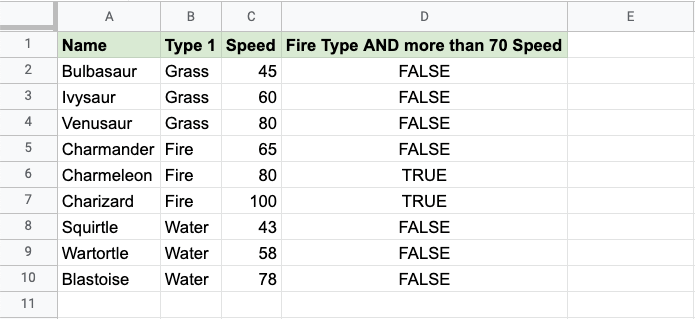Only Charmeleon and Charizard both have Fire type and speed greater than 70, so the function returns "TRUE".

## Example AND Function (with IF)

Combining the AND function with an IF function lets you check multiple conditions for the IF function:

Note: The IF function lets you specify the return values.

The IF function is typed `=IF` and has 3 parts:

=IF(logical_expression, value_if_true, value_if_false)

The AND function takes the place of the `logical_test` condition.

Here, the IF function returns "Yes" or "No".

Example AND function as the condition for IF function, step by step:

1. Select cell `C2`
2. Type `=IF`
3. Click the IF command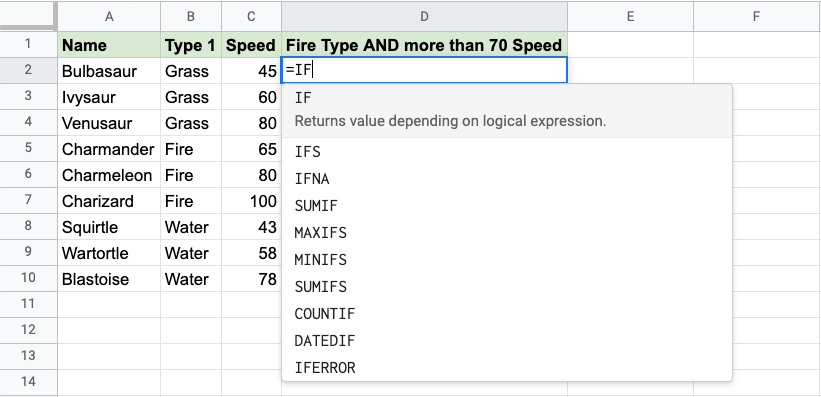1. Type `AND`
2. Click the AND command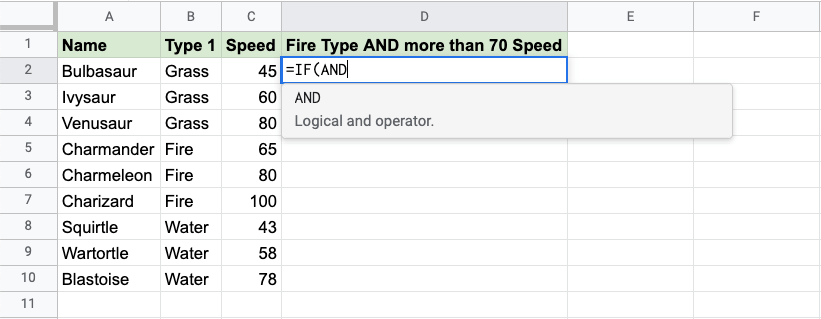1. Specify the first condition `B2="Fire"`
2. Type `,`
3. Specify the second condition `C2>70`
4. Type `),`
5. Specify the value `"Yes"` for when both conditions are TRUE
6. Type `,`
7. Specify the value `"No"` for when either, or both, conditions are FALSE
8. Type `)` and hit enter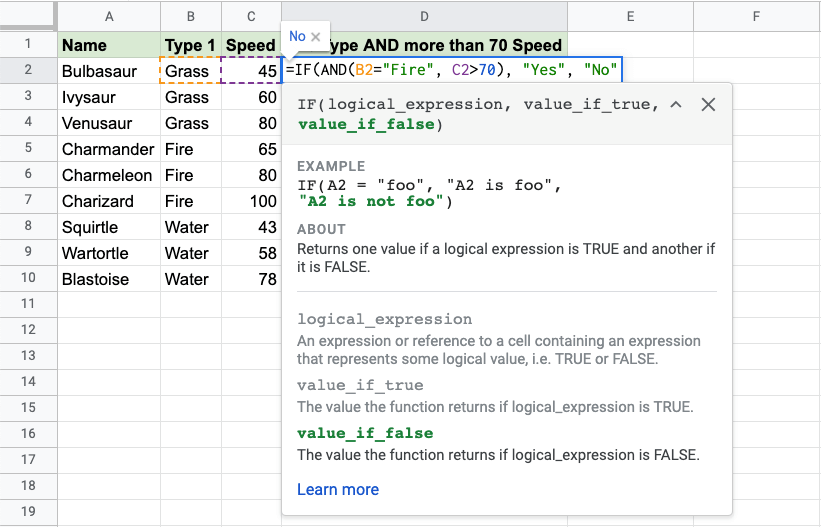The function can be repeated with the filling function for each row to perform the same check for each Pokemon:Now each row has a check for both being Fire type and Speed greater than 70: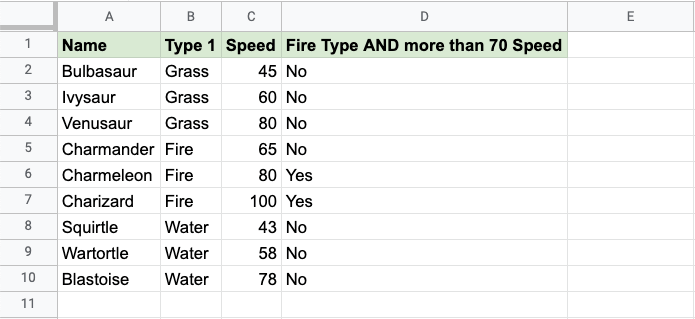Only Charmeleon and Charizard both have Fire type and speed greater than 70, so the function returns "Yes".

W3Schools is optimized for learning and training. Examples might be simplified to improve reading and learning. Tutorials, references, and examples are constantly reviewed to avoid errors, but we cannot warrant full correctness of all content. While using W3Schools, you agree to have read and accepted our terms of use, cookie and privacy policy.